Next: 4.4 The paramagnet at fixed temperature Previous: 4.2 The Partition Function

# 4.3 Entropy, Helmholtz Free Energy and the Partition Function

Take-home message: Once we have the Helmholtz free energy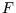we can calculate everything else we want.

Here is the crucial equation which links the Helmholtz free energy and the partition function: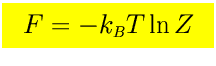The details of the derivation can be found here.

Since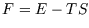, from the fundamental thermodynamic relation we obtain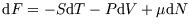. Thus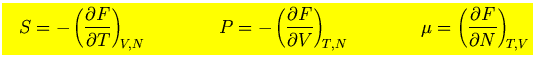(We first met these in the derivation of Maxwell's relations.) For a magnetic system, we have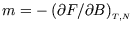instead of the equation for P.

Remember,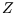and hencedepend on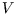(or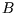) through the energies of the microstates. For instance the energy levels of a particle in a box of side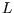are proportional to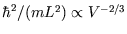.

These relations are reminiscent of those we met in the case of an isolated system, but there the entropy was the key; here it is the Helmholtz free energy. We can make the following comparison: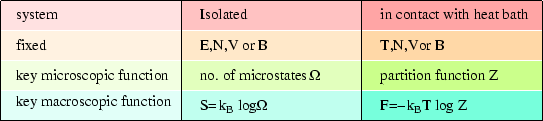It should not surprise us to find that the Helmholtz free energy is the key to a system at fixed temperature (in contrast to the entropy for an isolated system) as that is what we found classically (see here.)

References

• Mandl 2.5
• (Bowley and Sánchez 5.3-6)
• Kittel and Kroemer 3

SubsectionsNext: 4.4 The paramagnet at fixed temperature Previous: 4.2 The Partition Function
Judith McGovern 2004-03-17